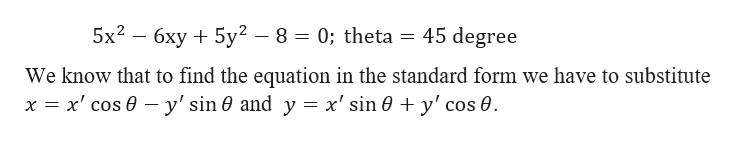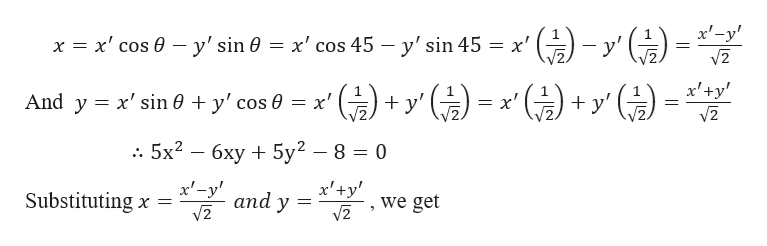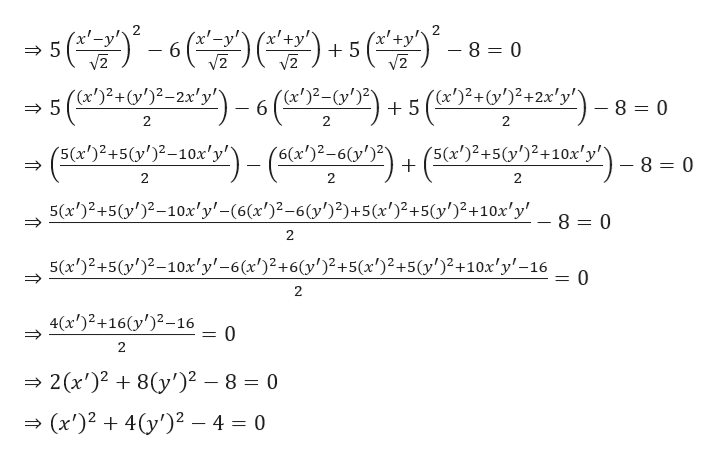# Write the equation in terms of a rotated x'y'- system using theta, the angle of the rotation. Write the equation involving x'and y' in standard form.5x2-6xy+5y2-8=0; theta=45 degree

Question
40 views

Write the equation in terms of a rotated x'y'- system using theta, the angle of the rotation. Write the equation involving x'and y' in standard form.

5x2-6xy+5y2-8=0; theta=45 degree

check_circle

Step 1

Given,help_outlineImage Transcriptionclose5x2 6xy5y2 - 8 = 0; theta = 45 degree We know that to find the equation in the standard form we have to substitute x x' cos ey' sin 0 and y = x' sin 0 + y' cos 0 fullscreen
Step 2

Now,help_outlineImage Transcriptionclosex-y х'-у' - y' sin 0 = x' cos 45 - y'sin 45 x' cos x "(y And y x'sin 0 y' cos 0 x' y' = x 6xy 5y2 8 = 0 .. 5x2 x-y Substituting x = x'+y and y we get fullscreen
Step 3help_outlineImage Transcriptionclose5 (r'?+cv'}2-2«*y") -6(«")> -6y°F + 5 ()2+")42x*y') - 8 = 0 2 (x'+y' 2 x'- - 8 = 0 6 V2 +2x'y 5 1 2 2 2 (5(x')2+5(y')2+10x'y +10X 80 (5(2+5()2-10x'y'-()-6 + = 2 2 5(x')2+5(y')2-10x'y(6(x')-6(y)2)+5(x')2+5(y')2+10x'y 8 0 2 5(x')2+5(y')2-10x'y'-6 (x')2+6(y')2+5(x')2+5(y')2+10x'y'-16 0 2 4(x')2+16y)2-16 _ 0 2 2(x')28y')2 - 8 = 0 (x')24y')2 - 4 = 0 fullscreen

### Want to see the full answer?

See Solution

#### Want to see this answer and more?

Solutions are written by subject experts who are available 24/7. Questions are typically answered within 1 hour.*

See Solution
*Response times may vary by subject and question.
Tagged in

### Calculus